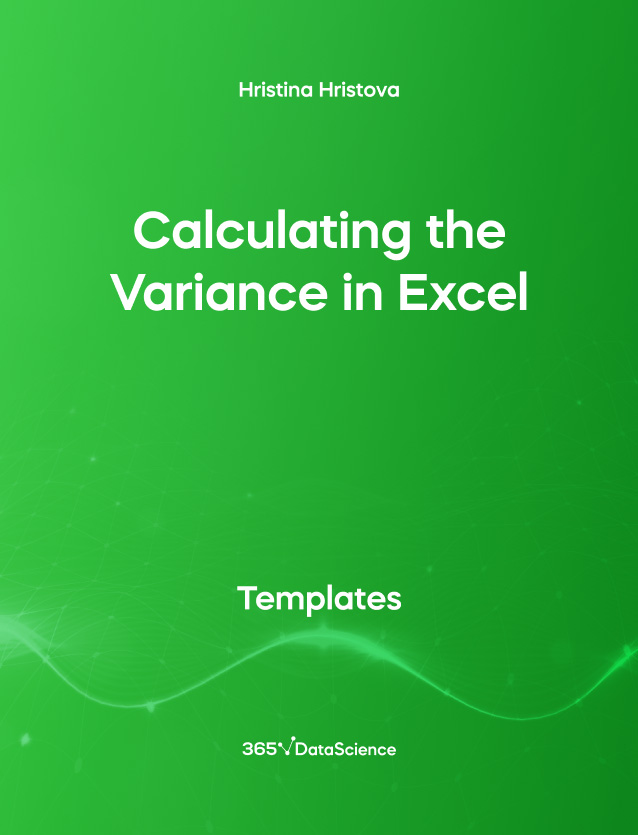## Calculating the Variance in Excel Template

The following Calculating the Variance in Excel template demonstrates the difference between sample variance and population variance. It introduces the relevant Excel syntax for calculating these 2 metrics. Some other related topics you might be interested in are Standard Deviation in Excel, Coefficient of Variation in Excel, Covariance in Excel, Correlation in Excel. You can now download the Excel template for free. Calculating the Variance in Excel template is among the topics covered in detail in the 365 Data Science program.Course Author

## Who is it for

This is an open-access Excel template that is going to be very helpful for Data Scientists, Data Analysts, Business Analysts and anyone who wants to know how to use sample variance and population variance.Variance measures the dispersion of a set of data points around their mean. In this template, you will notice that the variance of a dataset, called Population, is different depending on whether we consider it as a sample, or as the entire population. If we instead imagine that Population is a sample from a population, called Imaginary population, then we can calculate the mean and population variance of this latter bigger dataset.

## Join 2M Students and Start Learning from the best

The 365 Data Science program is great for beginners. It gives in-depth knowledge and clears every concept. After every lecture, some exercises are given for students to complete for practice, and solutions also. I would highly recommend this program to everyone who wants to be a data scientist and brush up their skills.

harshita sharma Pakistan

The 365 Data Science course is outstanding, it has an outstanding interface and awesome courses from a basic to an advanced level. I really appreciate the quality of the material and the content of this Data Science course!! Thanks to the 365 Data Science team!

Rakesh Patel United States

The 365 Data Science program was excellent. It does meet my expectations 100%. I do thank the 365 Data Science Team for putting this great work together in one package & with a very reasonable price. Hands down, you are the best! Please, keep this great work up!

Amrouni France

### Courses where you'll use this resource